ELASTIC CONSTANTS BASICS
Well logs are often used to determine the mechanical properties of rocks. These properties are often called the elastic properties or elastic constants of rocks. The subject matter and practice of calculating these rock properties is often called "rock physics".

The best known elastic constants are the bulk modulus of compressibility, shear modulus, Young's Modulus (elastic modulus), and Poisson's Ratio. The dynamic elastic constants can be derived with appropriate equations, using sonic log compressional and shear travel time along with density log data.

Elastic constants are needed by five distinct disciplines in the petroleum industry:
1. geophysicists interested in using logs to improve synthetic seismograms, seismic models, and interpretation of seismic attributes, seismic inversion, and processed seismic sections.
2. production or completion engineers who want to determine if sanding or fines migration might be possible, requiring special completion operations, such as gravel packs
3. hydraulic fracture design engineers, who need to know rock strength and pressure environments to optimize fracture treatments
4. geologists and engineers interested in in-situ stress regimes in naturally fractured reservoirs
5. drilling engineers who wish to prevent accidentally fracturing a reservoir with too high a mud weight, or who wish to predict overpressured formations to reduce the risk of a blowout.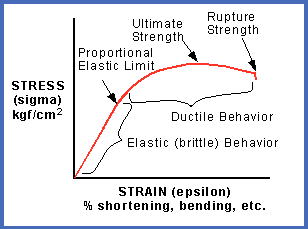Mechanical properties are used to design hydraulic fracture stimulation programs in oil and gas wells, and in the design of mines and gas storage caverns. In this situation, the mechanical properties are derived in the laboratory or from well log analysis, calibrated to the lab results.

In seismic petrophysics, these same mechanical properties are called seismic attributes. They are derived by inversion of time-domain seismic data, calibrated to results from well log analysis, which in turn were calibrated to the lab data. The vertical resolution of seismic data is far less than that of well logs, so some filtering and up-scaling issues have to be addressed to make the comparisons meaningful.

The main purpose for finding  these attributes is to distinguish reservoir quality rock from non-reservoir. The ultimate goal is to determine porosity, lithology, and fluid type by "reverse-engineering" the seismic attributes. The process is sometimes called "quantitative seismic interpretation". In high porosity areas such as the tar sands, and in high contrast areas such as gas filled carbonates,, modest success has been achieved, usually after several iterative calibrations to log and lab data. Something can be determined in almost all reservoirs, but how "quantitative" it is may not be known.

There are many other types of seismic attributes related to the signal frequency, amplitude, and phase, as well as spatial attributes that infer geological structure and stratigraphy, such as dip angle, dip azimuth, continuity, thickness, and a hundred other factors. While logs may be used to calibrate or interpret some of these attributes, they are not discussed further here.ELASTIC CONSTANTS DEFINITIONS
The elastic constants of rocks are defined by the Wood-Biot-Gassmann Equations. The equations can be transformed to derive rock properties from log data. If crossed dipole sonic data is available, anisotropic stress can be noticed by differences in the X and Y axis displays of both the compressional and shear travel times. When this occurs, all the elastic constants can be computed for both the minimum and maximum stress directions. This requires the original log to be correctly oriented with directional information, and may require extra processing in the service company computer center.

Elasticity is a property of matter, which causes it to resist deformation in volume or shape. Hooke's Law, describing the behavior of elastic materials, states that within elastic limits, the resulting strain is proportional to the applied stress. Stress is the external force applied per unit area (pressure), and strain is the fractional distortion which results because of the acting force.The modulus of elasticity is the ratio of stress to strain:
0: M = Pressure / Change in Length =  {F/A} / (dL/L)

This is identical to the definition of Young's Modulus. Both names are used in the literature so terminology can be a bit confusing.

Different types of deformation can result, depending upon the mode of the acting force. The three elastic moduli are:

Young's Modulus Y (also abbreviated E in various literature),
1: Y = (F/A) / (dL/L)

Bulk Modulus Kc,
2: Kc = (F/A) / (dV/V)

Shear Modulus N, (also abbreviated as u (mu))
3: N = (F/A) / (dX/L) = (F/A) / tanX

Where:
F/A = force per unit area
dL/L, dV/V, dX/L = fractional strains of length, volume, and shape, respectively.
Note: dX/L  can be represented by tanX.Poisson's Ratio PR (also abbreviated v (nu)), is defined as the ratio of strain in a perpendicular direction to the strain in the direction of extensional force,
4: PR = (dX/X) / (dY/Y)

Where:
X and Y = original dimensions
dX and dY = changes in X and Y directions respectively, as
the deforming stress acts in Y direction.

Young's Modulus vs Poison's Ratio: Brittleness increases toward top left, density increases toward top right, porosity plus organic content and depth decrease toward bottom left. PR values less than 0.17 indicate gas or organic content or both. (image courtesy Canadian Discovery Ltd)

All of these moduli can be derived directly from well logs and indirectly from seismic attributes:
5: N = KS5 * DENS / (DTS ^ 2)
6: R = DTS / DTC
7: PR = (0.5 * R^2 - 1) / (R^2 - 1)
8: Kb = KS5 * DENS * (1 / (DTC^2) - 4/3 * (1 / (DTS^2)))
9: Y = 2 * N * (1 + PR)

Lame's Constant Lambda, (also abbreviated λ) is a measure of a rocks brittleness, which is a function of both Young's Modulus and Poisson's Ratio:
10:
Lambda = Y * PR / ((1 + PR) * (1 - 2 * PR))
OR 10A: Lambda = DENS * (Vp^2 - 2 * Vs ^ 2)

Some people prefer different abbreviations: Mu or u for shear modulus, Nu or v for Poisson's Ratio, and E for Young's Modulus. The abbreviations used above are used consistently trough these training materials.

In the seismic industry, it is common to think in terms of velocity and acoustic impedance in addition to the more classical mechanical properties described above.

The compressional to shear velocity ratio is a good lithology indicator:
11. R = Vp / Vs = DTS / DTC

Acoustic impedance:
12: Zp = DENS / DTC
13: Zs = DENS / DTS

Where:
DTC = compressional sonic travel time
DTS = shear sonic travel time
DENS = bulk density
KS5 = 1000 for metric units

An example of a log analysis for mechanical rock properties (elastic constants) is shown below. Coloured dots represent lab derived data, and illustrate the close match obtained betwee log analysis and lab measured data.Dynamic elastic properties calculated from density and sonic log data, showing close match to dynamic data from lab measurements (coloured dots). Lab data is from table shown above. Note synthetic sonic and density plotted next to measured log curves (Tracks 2 and 3), showing reasonably small differences due to minor borehole effects. Synthetic curves can repair worse logs or even replace missing curves.ELASTIC CONSTANTS CROSSPLOTS
Composite seismic attributes, such as Lame's Constant times density (Lambda_Rho) and shear modulus times density (Mu_Rho), are used to normalize attributes to make interpretation easier. Various crossplots of results are used to distinguish differences between rock types, as shown below. The colour code represents depth (red-orange = shallower, blue-green = deeper)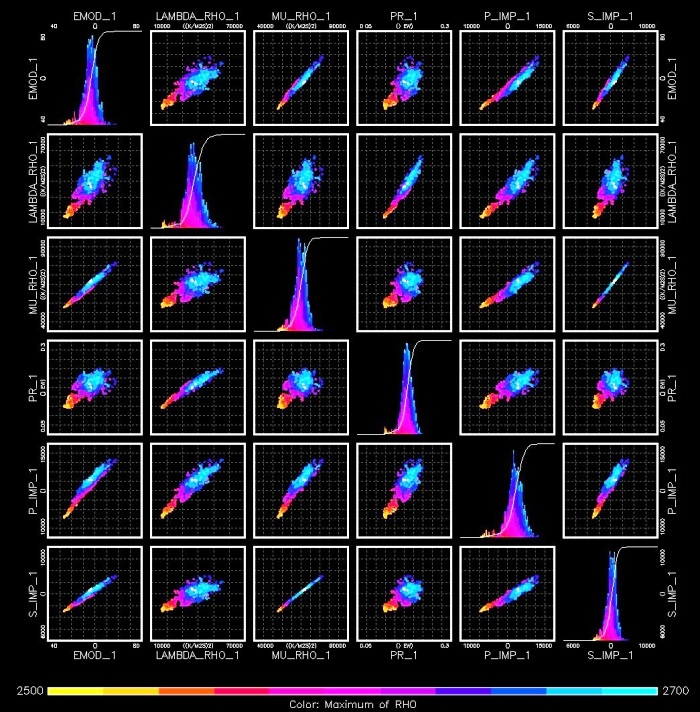Crossplots of the elastic constants are used to identify variations in rock characteristics, by noting changes in the data distributions. (RHO = density, PR = Poisson's Ratio, MU = shear Modulus, LAMBDA = Lame's Constant, BMOD = bulk modulus, EMOD = Young's Modulus, P_IMP = compressional wave acoustic impedance, S_IMP = shear wave acoustic impedance, (image courtesy Canadian Discover Ltd)EFFECTS OF ANISOTROPY
The elastic constants are often considered to be uniform in the three cardinal axes. Under this assumption, a sonic log would read the same value in all directions. However, in a rock under horizontal tectonic stress, there is a minimum and a maximum stress direction, and the acoustic properties vary with that stress. Using the crossed dipole mode of the dipole shear sonic log, we can provide acoustic velocity (or travel time) in these two directions.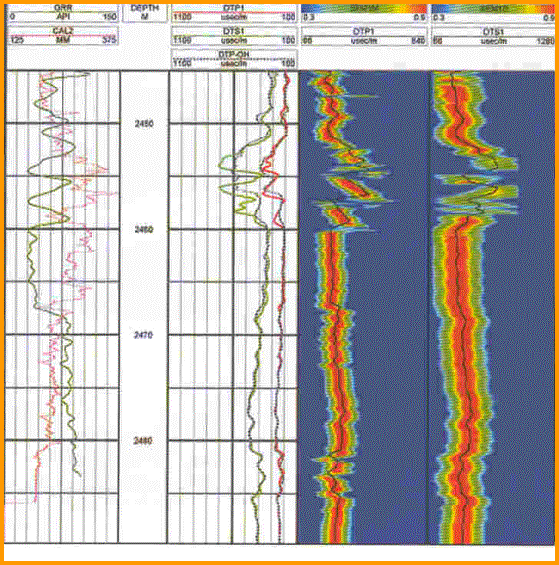Example of a dipole shear image log run in crossed dipole mode. It has two compressional and two shear curves measured in orthogonal directions. An anisotropy coefficient can be computed from the difference between the two compressional curves - note the interval near the top f the log where the curves separate and the image log gets "shaky", indicating stress anisotropy. Fractures are indicated where the high amplitude (red colour on image log) disappears.

Full wave, array, and dipole sonic log presentations vary widely, depending on age, service company, and intended use.

Acoustic anisotropy coefficient is defined as:
1: Kani = 0.5 * (Vmax - Vmin) / (Vmax + Vmin)

Where:
Kani = anisotropy coefficient (fractional)
Vmin = minimum acoustic (seismic) velocity (m/sec or ft/sec)
Vmax = maximum acoustic (seismic) velocity (m/sec or ft/sec)

Equation 1 can be rewritten in log analysis terms as:
2: Kani = 0.5 * (DTCmax - DTCmin) / (DTCmax + DTCmin)

Because of tool rotation, the log curves that represents the maximum and minimum values trade places, so the best solution is to take the absolute value of the difference between the two sonic log curves in the numerator. Some people multiply the anisotropy coefficient by 100 and display it as a percentage. Typical values range from zero for no anisotropy to as much as 25% in highly stressed regions.

An anisotropy coefficient based on resistivity values is also generated from well logs, but it has nothing to do with stress assessments. In this case it refers to the differences between vertical and horizontal resistivity in laminated rocks.:
3: AnisRatio = RESvert / REShoriz
4: AnisCoef = AnisRatio ^ 0.5

The resistivity ratio as defined here is nearly always greater than 1.0, and some literature uses the inverse of these terms, maintaining the same nomenclature..LAB MEASUREMENT PROCEDURES
Elastic properties are measured in the laboratory using triaxial stress tests (static measurements) and by measuring bulk density and acoustic travel time with a high frequency impulse (dynamic testing). Both are done under representative overburden pressure.

The general procedures for triaxial compressive test are:
1. A right cylindrical plug is cut from the sample core and their ends ground parallel according to International Society for Rock Mechanics (ISRM) and American Society for Testing and Materials (ASTM) standards.  A length to diameter ratio of 2:1 is recommended to obtain representative mechanical properties of the sample, which is also recommended by ASTM and ISRM.  Physical dimensions and weight of the specimen are recorded and the specimen is saturated with simulated formation brine.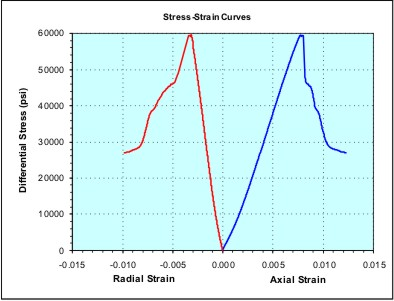2. The specimen is then placed between two plates and a heat-shrink jacket is placed over the specimen.

3. Axial strain and radial strain devices are mounted in the endcaps and on the lateral surface of the specimen, respectively.

4. The specimen assembly is placed into the pressure vessel and the pressure vessel is filled with hydraulic oil.

5. Confining pressure is increased to the desired hydrostatic testing pressure.

6. Measure ultrasonic velocities at the hydrostatic confining pressure.

7. Specimen assembly is brought into contact with a loading piston that allows application of axial load.

8. Increase axial load at a constant rate until the specimen fails or axial strain reaches a desired amount of strain while confining pressure is held constant.

9. Reduce axial stress to the initial hydrostatic condition after sample fails or reaches a desired axial strain.

10. Reduce confining pressure to zero and disassemble sample.

 Depth (m) Confining Pressure (psi) Compressive Strength (psi) Static Young's Modulus (x106 psi) Static Poisson's Ratio XX51.50 3850 63359 8.70 0.40 XX61.15 3850 56831 5.75 0.36 XX71.15 3850 56026 5.79 0.34 XX05.20 3850 50910 5.08 0.39

Static elastic properties measured with triaxial stress test

 Depth Bulk Ultrasonic Wave Velocity Dynamic Elastic Parameter m Density g/cc Compressional ft/sec  usec/ft Shear ft/sec usec/ft Young's Modulus (x106 psi) Poisson's Ratio Bulk Modulus (x106 psi) Shear Modulus (x106 psi) XX51.50 2.81 20161 49.60 10760 92.94 11.39 0.30 9.53 4.38 XX61.15 2.57 15829 63.18 9555 104.66 7.68 0.21 4.46 3.16 XX71.15 2.66 17226 58.05 10299 97.10 9.30 0.22 5.57 3.81 XX05.20 2.64 16451 60.79 9763 102.43 8.31 0.23 5.10 3.38

Dynamic elastic properties measured with ultrasonic impulse in the lab. Note differences between static and dynamic values. Elastic properties from log analysis models match lab dynamic data better than static data.Dynamic elastic properties calculated from density and sonic log data, showing close match to dynamic data from lab measurements (coloured dots). Lab data is from table shown above. Note synthetic sonic and density plotted next to measured log curves (Tracks 2 and 3), showing reasonably small differences due to minor borehole effects. Synthetic curves can repair worse logs or even replace missing curves.Examples of Mechanical Properties Logs
The format and curve complement of Mechanical Properties Logs vary widely between service companies and age of log. Some logs have Metric depths but the moduli are in English units. Some are vice versa. Here are some examples.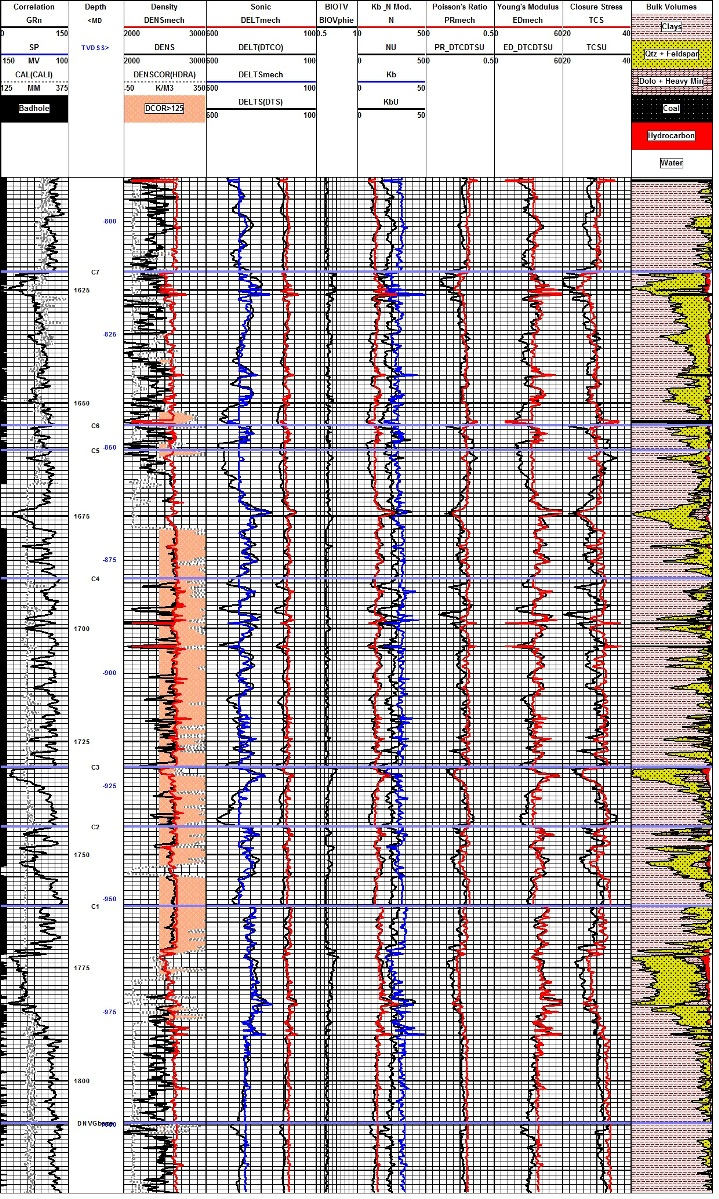Example of log reconstruction in a shaly sand sequence (Dunvegan). The 3 tracks on the left show the measured gamma ray, caliper, density, and compressional sonic. Original density and sonic are shown in black, modeled logs are in colour. Shear sonic is the model result as none was recorded in this well. Computed elastic properties are shown in the right hand tracks. Results from the original unedited curves are shown in black, those after log editing are in colour. Note that the small differences in the modeled logs compared to the original curves propagate into larger differences in the results, especially Poisson's Ratio (PR), Young's Modulus (ED), and total closure stress (TCS).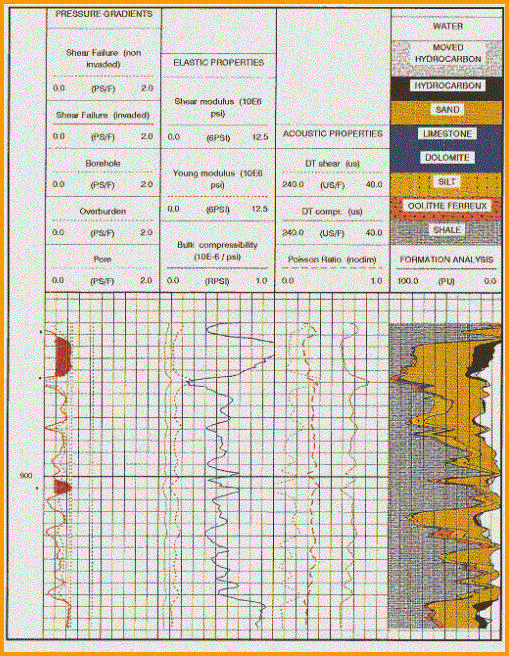Mechanical properties log with lithology/porosity track at the right. This analysis was run to find out if sanding might occur during production from the oil zone. High bulk modulus and low sher modulus suggest sanding is like. Stress failre (shaded black in Track 1) shows where sanding is most likely to occur.

Page Views ---- Since 01 Jan 2015
Copyright 2023 by Accessible Petrophysics Ltd.
CPH Logo, "CPH", "CPH Gold Member", "CPH Platinum Member", "Crain's Rules", "Meta/Log", "Computer-Ready-Math", "Petro/Fusion Scripts" are Trademarks of the Author